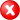#Boost C++ Libraries

...one of the most highly regarded and expertly designed C++ library projects in the world.

##### centroid (with strategy)

Calculates the centroid of a geometry using the specified strategy.

###### Description

The free function centroid calculates the geometric center (or: center of mass) of a geometry. Reasons to specify a strategy include: use another coordinate system for calculations; construct the strategy beforehand (e.g. with the radius of the Earth); select a strategy when there are more than one available for a calculation.

###### Synopsis

```template<typename Geometry, typename Point, typename Strategy>
void centroid(Geometry const & geometry, Point & c, Strategy const & strategy)```

###### Parameters

Type

Concept

Name

Description

Geometry const &

Any type fulfilling a Geometry Concept

geometry

A model of the specified concept

Point &

Any type fulfilling a Point Concept

c

A model of the specified Point Concept which is set to the centroid

Strategy const &

Any type fulfilling a Centroid Strategy Concept

strategy

The strategy which will be used for centroid calculations

Either

`#include <boost/geometry.hpp>`

Or

`#include <boost/geometry/algorithms/centroid.hpp>`

###### Conformance

The function centroid implements function Centroid from the OGC Simple Feature Specification.

###### Behavior

Case

Behavior

Point

Returns the point itself as the centroid

Multi Point

Calculates centroid (based on average)

linear (e.g. linestring)

Calculates centroid (based on weighted length)

areal (e.g. polygon)

Calculates centroid

Empty (e.g. polygon without points)

Throws a centroid_exception

Cartesian

Implemented

Spherical

Calculates the centroid as if based on Cartesian coordinates

###### Supported geometries

2D

3D

PointSegmentBoxLinestringRingPolygonMultiPointMultiLinestringMultiPolygonLinear